## 1. Introduction

In this tutorial, we describe how to use the silhouette plot in cluster analysis.

Clustering is one of the unsupervised learning methods. First, we explain what silhouette values measure and how to calculate and interpret them. Then, we show how to determine the number of clusters using the mean silhouette value.

## 2. Silhouette Plots in Cluster Analysis

A silhouette plot is a graphical tool depicting how well our data points fit into the clusters they’ve been assigned to. We call it the quality of fit cohesion.

At the same time, a silhouette plot shows the quality of separation: this metric conveys the degree to which the points that don’t belong to the same cluster have been assigned to different ones.

To analyze clusters, we need to consider both criteria, which silhouette plots allow us to do.

## 3. Silhouette Values

A silhouette value is a combination of two scores: cohesion and separation.

### 3.1. Cohesion

Cohesion measures the similarity of the points in the same cluster. So, we can call it an intra-cluster metric.

Letbe a cluster andtwo points in it. Then, we can interpret the distance between them as a measure of their similarity. From there, we define the cohesion of pointin its clusteras the mean distance betweenand the other pointsin:### 3.2. Separation

On the other hand, separation refers to the degree to which the clusters don’t overlap. So, it’s an inter-cluster metric.

Intuitively, the distance between the clusters speaks about the “goodness of their separation”. So, we define the separation ofas the minimum mean distance betweenand other clusters:### 3.3. Combining Cohesion and Separation into a Silhouette Value

Then, the silhouette value of a pointis:Its range is. The higher the silhouette value, the more certain we can be that its label is correct. So, a high mean silhouette value of all the points indicates a good clustering.

### 3.4. How to Calculate the Silhouette Value?

Let’s take a look at clusters, andin the following image: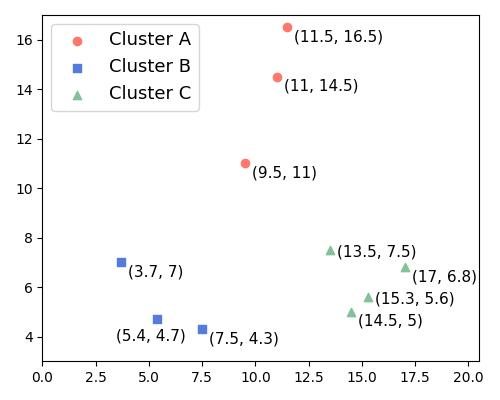Let’s compute the silhouette value of the point. To do so, we need to calculate its cohesion and separation scores.

The cohesion of pointis its mean distance to the other points in. The distance between the pointand pointis:and the distance tois:So, the cohesion of pointis:We can compute the mean distance of the pointto clusterin the same way:Following the same steps, we compute its mean distance to cluster:Therefore, the separation score ofis, since that’s the lower of the two values.

From there, we get the silhouette value of:Sinceis close to the theoretically maximal silhouette value, we can be confident that we assignedto the cluster it actually belongs to.

### 3.5. Analyzing the Silhouette Values

Let’s say that we have two clusters:and, such thatis the cluster closest to. We’re calculating the silhouette values of the points in. The silhouette valueof any point inwill be closer to 1 when. In that case, the distance betweenand the other points inis much smaller than the distance to points in. This indicates thatbelongs to cluster: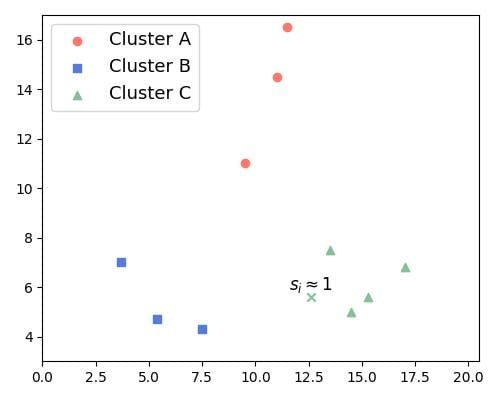Therefore, as the average of the silhouette values of the points in a cluster gets closer to 1, the cohesion and separation of the cluster as a whole increase.

The converse is also true. When the silhouette value is closer to zero, bothandare similar. Then, the distances betweenand the elements onandare similar. Thus, it is not clear ifshould belong toor: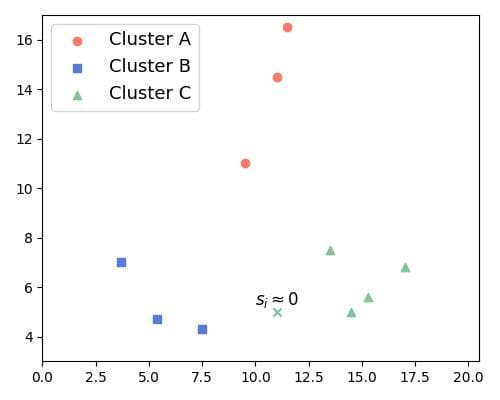The worst case occurs when the silhouette valueis negative, which happens whenis greater than. Then, the point in question lies closer tothan to. So, it looks misclassified: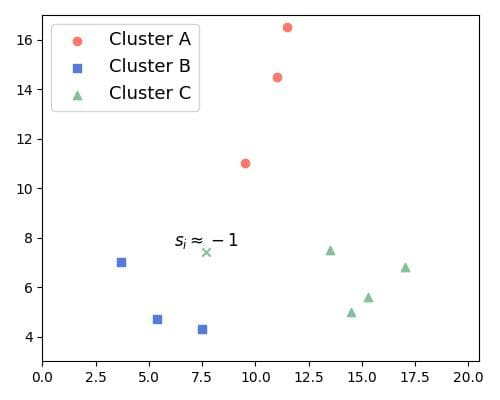Hence, as thevalues decrease, the quality of separation deteriorates, and the quality of the clustering gets worse.

## 4. Silhouette Plots

The silhouette of a cluster visualizes the silhouette valuesof all the points in it in the decreasing order. A silhouette plot shows the silhouettes of all the clusters in random order. Additionally, it inserts blank spaces between consecutive clusters and can color them differently.

For example, here’s a plot for four clusters we got with the K-Means clustering algorithm on an ad-hoc two-dimensional dataset: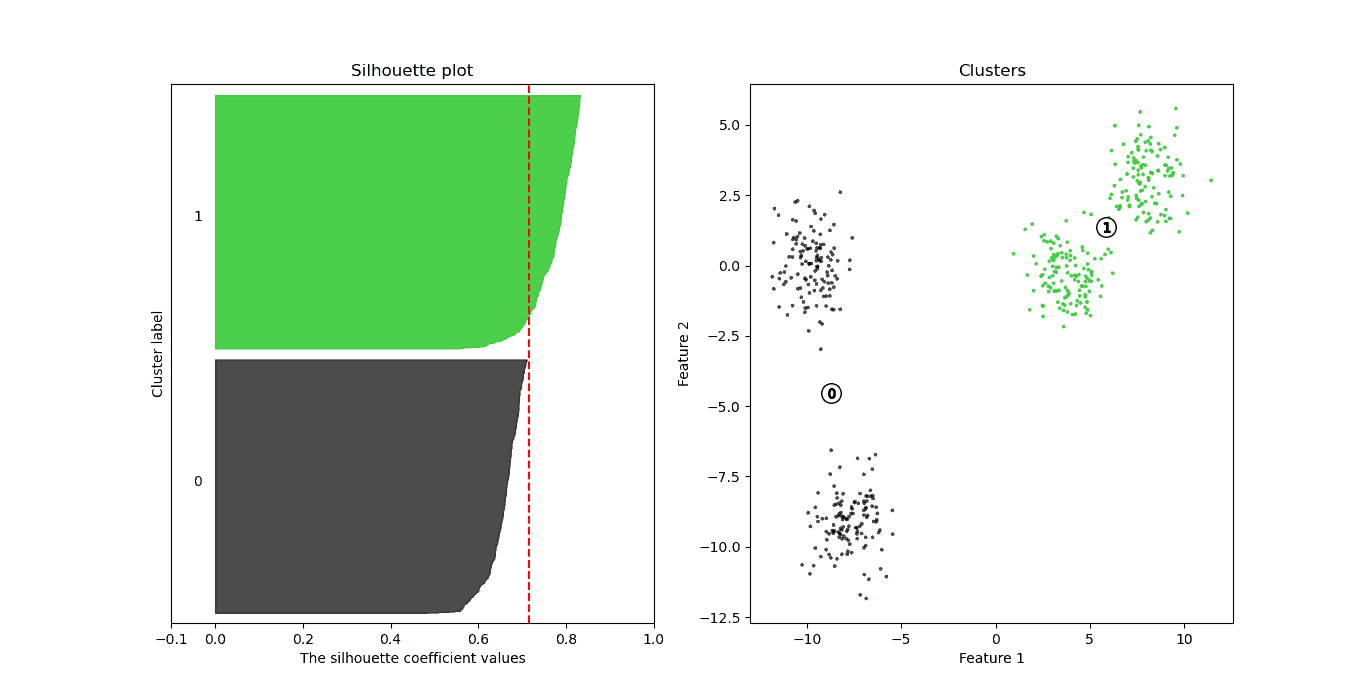Here, we set. On the left, we have the silhouette plot. The-axis shows the silhouette values, and the height of each silhouette indicates the number of points in the corresponding cluster. The right subplot visualizes the data points with the same colors as the clusters. The red line shows the average silhouette value for all the clusters. In this example, the average value is 0.71.

From the right subplot, we conclude that the cohesion of the green points is higher than that of the black ones. This should explain the worse silhouette of the black cluster on the left. However, the two clusters look well separated.

## 5. Choosing the Number of Clusters

By plotting the silhouettes for different values of, we can see whichbest fits the data.

For instance, the following graphic shows the K-Means results for the above data and. The average silhouette value increases to 0.78: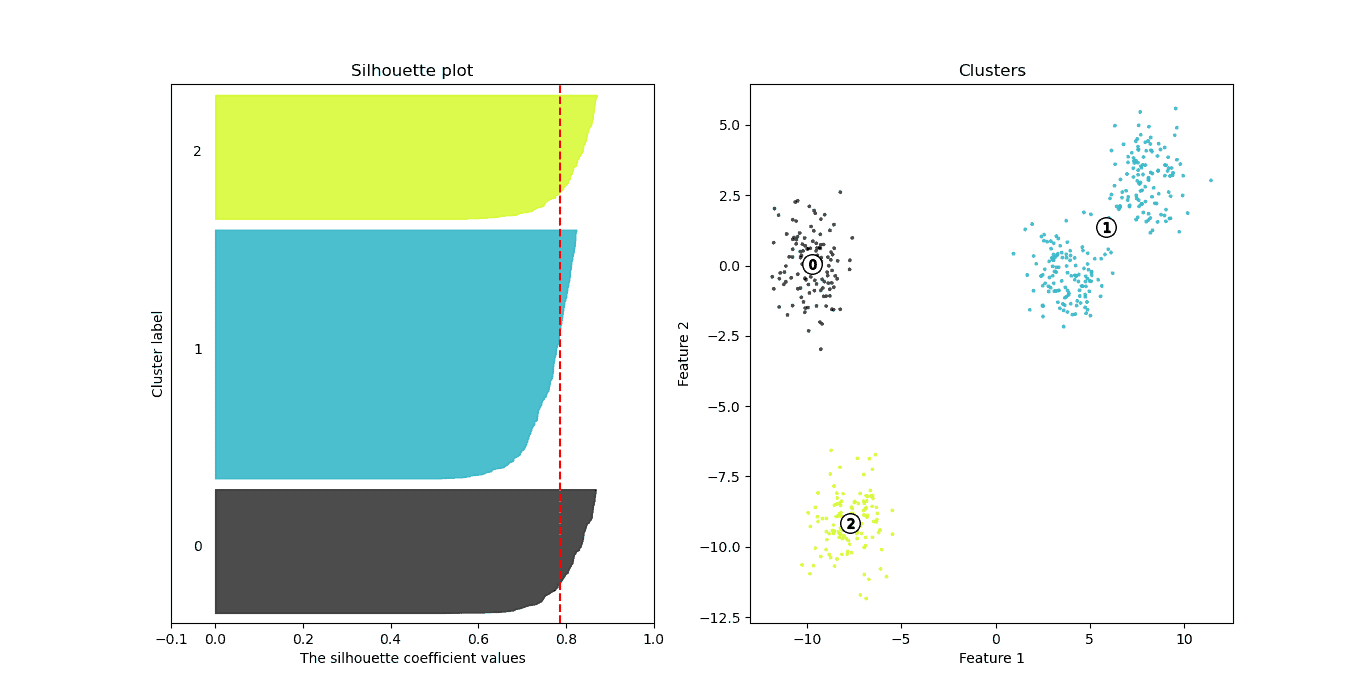Here, we see that the right cluster remains intact, while the left one splits into two smaller ones. These two clusters have better silhouette values than the ones obtained by the blue cluster, but all three appear to be well defined.

What happens if we use? The average silhouette value drops slightly to 0.74: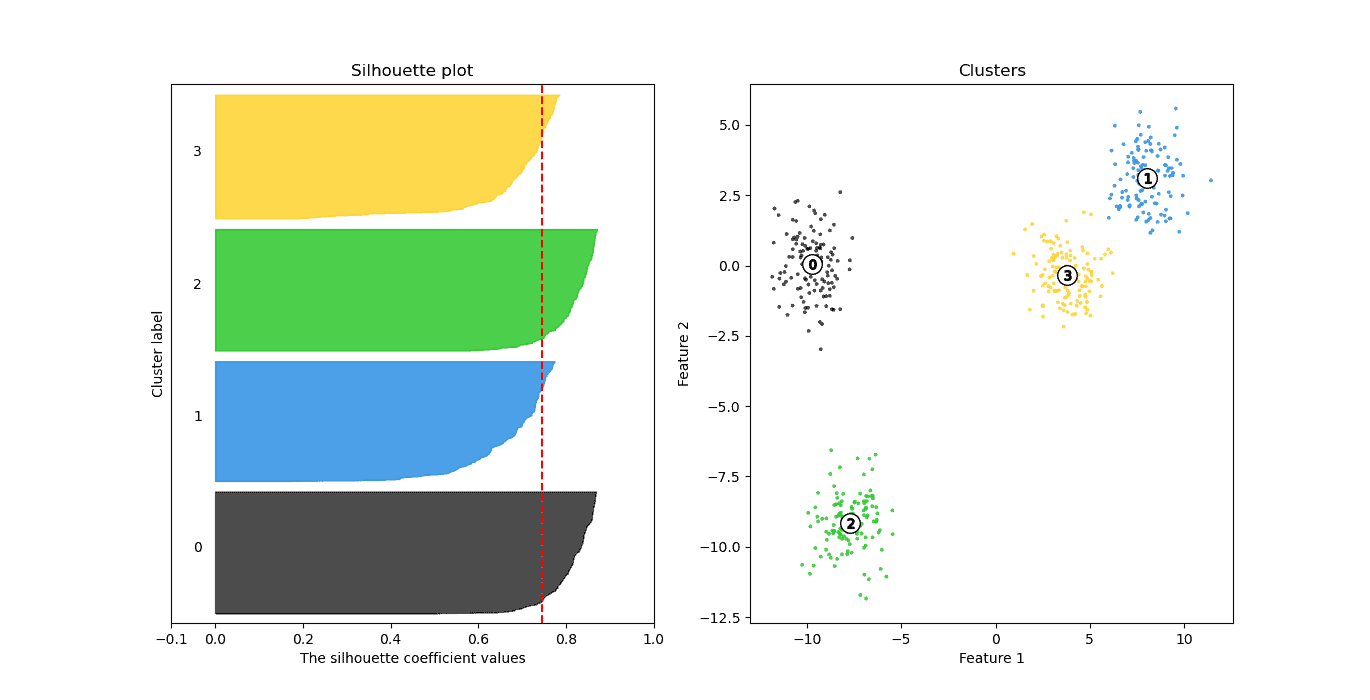Here, we see that the green and black clusters have better silhouettes than the other two. It’s probably because their points are better separated than the points of the other two clusters.

Finally, the average silhouette values fordrops to 0.66 and 0.53: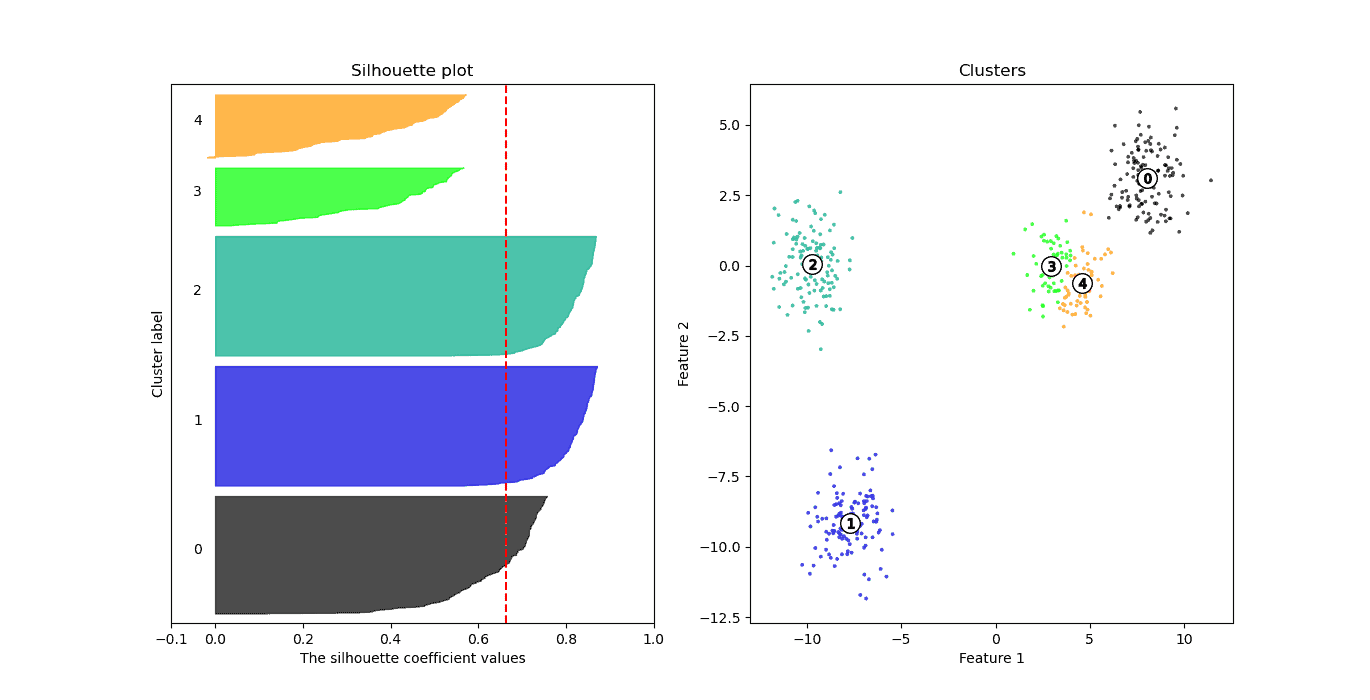and forto 0.53: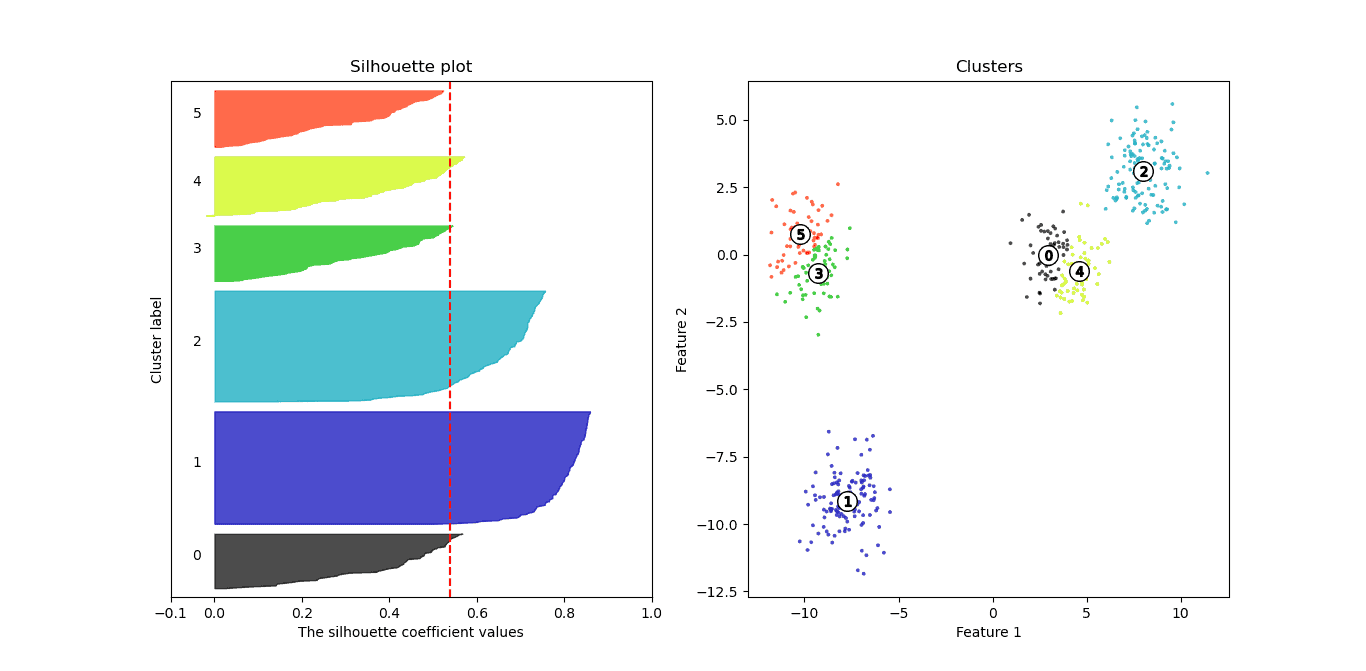So,appear to be good choices, whereasandgive lower-quality clusters.

### 5.1. Interpreting the Mean of Silhouette Values

Silhouette values measure the relation between cluster cohesion and cluster separation. Thus, the mean of the silhouette values represents the balance of the overall cohesion and separation in all the clusters.

As we concluded previously, the cohesion and separation of clusters are better when the silhouette values are close to 1. Therefore, we’re looking for the clustering with a higher mean silhouette value, ideally close to 1.

One way to select the number of clusters could be to choose the one with the higher overall mean. Kaufmann and Rousseeuw (1990) named the overall mean the silhouette coefficient (SC). By their classification, if> 0.70, the structure of the clusters is strong. Ifis between 0.51 and 0.70 the structure is reasonable. Lower values indicate poor structure.

Here are the mean values forwe got in the above example:Considering thecriterion, acceptable solutions are those with 2, 3, and 4 clusters. In this particular simulation, we used the data from four blobs, so the best solution should be the one with 4 clusters. But, we can see that the solution with 3 clusters looks even better.

## 6. Conclusion

In this tutorial, we talked about silhouette plots and values. A silhouette plot is a graphical tool we use to evaluate the quality of clusters. The silhouette values show the degree of cohesion and separation of the clusters. The mean of the silhouette values allows identifying how many clusters appear in the dataset.